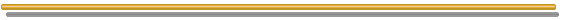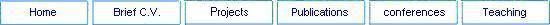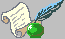Publications[Books Papers]List Of Publications

## Books

1.  Sampling Methods, Dar Al-Forqan, Amman, Jordan (1993).

2.  Lab Manual for Statistics for Engineers and Scientists, KFUPM,  2004.

3. Sampling Methods and Data Collection, Obeikane Publisher, Riyadh, KSA (2010).

4. Ranked Set Sampling, Cambridge Scholars Publishing, (with Ahmad, M. and Hanif Editors), UK (2010).

5.  Introduction to Statistical Methods, in process.

6.  Sampling Methods in process.

1. Ranked Set Sampling with Respect to Concomitant Variables and with Size Biased Probability of Selection (with McDonald, L.)Communication in Statistics Theory and Methods. Vol. 19 (1990), pp. 205-219.

2. Ranked Set Sampling with Size Biased Probability of Selection (with L. McDonald), Biometrics. Vol. 46 (1990), pp. 435-445.

3. Ranked Set Sampling and Line Intercept Method: A More Efficient Procedure (with L. McDonald), Biometrical Journal. Vol. 34 (1992), pp. 329-346.

4. A Note on Line Intercept Method (with S. Sadooghi- Alvandi), Biometrics. Vol. 49 (1993), pp. 1209-1215.

5. Parameters Estimation in a Simple Linear Regression using Ranked Set Sampling, Biometrical Journal. Vol. 37 (1994), pp. 799-810.

6. Combining the Line Intercept Sampling Method with the Ranked Set Sampling, Journal of Information & Optimization Sciences. Vol. 16 (1995), pp. 1-16.

7. Encounter Sampling with Line Intercept Sampling Method, Journal of Information & Optimization Sciences. Vol. 16 (1995), pp.417-427.

8. Utilizing some Available Prior Information in Estimating the Mean of a Normal Population: A Sequential Approach (with M. Al-Saleh ), Pakistan Journal of Statistics. Vol. 11 (1995), pp. 99-109.

9. Using Ranked Set Sampling for Testing Hypotheses on the Scale Parameter for Exponential and Uniform Distributions, (W. with Abu-Dayyeh), Pakistan Journal of Statistics. Vol. 12 (1996), pp. 131-138.

10. Estimation of Parameters for One-Way Layout with Ranked Set Sampling, Biometrical Journal. Vol. 38 (1996), pp. 507-515.

11. Estimation of Parameters in a Multiple Regression Model using Ranked Set Sampling, Journal of Information & Optimization Sciences. Vol. 17 (1996), pp. 521-533.

12. Pair Ranked Set Sampling, Biometrical Journal. Vol. 38 (1996), pp. 879-885.

13. Estimation of Ratio using Ranked Set Sampling (with H. Samawi), Biometrical Journal. Vol. 38 (1996), pp. 753-764.

14. Median Ranked Set Sampling, Journal of Applied Statistical Sciences. 6 (1997), pp. 245-255.

15. A Note in Bayesian Estimation using Ranked Set Sampling (with M. Al-Saleh), Pakistan Journal of Statistics. Vol. 14 (1998), pp. 49-56.

16. MIVQUE and REML Estimators of Variance Components under Proportionality Condition (with S. Hossain), Biometrical Journal. Vol. 40 (1998), pp. 845-854.

17. Testing Hypotheses for the Normal Distribution using Ranked Set Sampling (with W. Abu-Dayyeh), Journal of Information & Optimization Sciences. Vol. 19 (1998), pp. 1-11.

18. Median Ranked Set Sampling: A Comparison with Ranked Set Sampling and Regression Estimators, Environmetrics. Vol. 9, (1998), pp. 255-267.

19. Median Ranked Set Sampling with Size Biased Probability of Selection, Biometrical Journal. Vol. 40 (1998), pp. 455-465.

20. Paired Ranked Set Sampling: A More Efficient Procedure (with S. Hossain), Environmetrics. Vol. 10 (1999), pp. 195-212.

21. Adaptive Cluster Sampling for Characteristics other than Total Number of Elements (with A. Khan and M. Bhatti), Parisankyan Samikkha. Vol. 6 (1999), pp. 19-32.

22. On Extreme Ranked Set Sampling with Size Biased Probability of Selection, Far East Journal of Theoretical Statistics. Vol. (1999), pp. 319-329.

23. Bayesian Estimation using Ranked Set Sampling  (with M. Al-Saleh and K. Al-Shrafa), Biometrical Journal. Vol. 42 (2000), pp. 489-500.

24. MVLUE of Population Parameters Based on Ranked Set Sampling (with S. Hossain), Applied Mathematics and Computation. Vol. 108 (2000), pp167-176.

25. Recent Developments on Ranked Set Sampling  (with M. Al-Saleh), Pakistan Journal of Statistics. Vol. 16 (2000), pp 269-290.

26. Testing Hypotheses about the Location Parameter of the Logistic Distribution using Simple Random Sampling and Ranked Set Sampling (with W. Abu-Dayyeh and  S. Al-Subh), Pakistan Journal of Statistics. Vol. 17 (2001), pp 37-50.

27. Selected Ranked Set Sampling (with S. Hossain), Australian & New Zealand Journal of Statistics. Vol. 43 (2001), pp 311-325.

28. Regression Estimators in Extreme and Median Ranked Set Sampling, Journal of Applied Statistics. Vol. 28, (2001), pp 1003-1017.

29. Extreme Ranked Set Sampling A Comparison with Regression and Ranked Set Sampling Estimator, Pakistan Journal of Statistics. Vol. 17 (2001), pp 187- 204.

30. Random Ranked Set Samples (with I. Rahimov), Pakistan Journal of Statistics. Vol. 17 (2001), 51-66.

31. On Ratio estimation using Median Ranked Set Sampling (with H. Samawi), Journal of Applied Statistical Sciences. Vol. 10 (2001), pp 89-99.

32. Variance Estimation using Ranked Set Sampling (with W. Abu-Dayyeh), Pakistan Journal of Statistics. Vol. 18 (2002).

33. Exact Bahadur Slope for Combining Independent Tests for Normal and Logistic Distributions (with W. Abu-Dayyeh and M. Al-Momani), Applied Mathematics and Computation. Vol. 135 (2002), pp. 135-150.

34. Adjusted two-stage Adaptive Cluster Sampling with Ranked Set Sampling (with A. Khan), The Bangladesh Journal of Scientific Research, (2002).

35. Adjusted Two-sage Adaptive Cluster Sampling (with A. Khan), Environmental and Ecological Statistics. Vol. 9 (2002), pp. 111-120.

36. A Comparison of the Maximum Likelihood Estimators under Ranked Set Sampling and some of its Modifications (with A. Khan), Applied Mathematics and Computation, Vol. 129 (2002), pp. 441- 453.

37. Some Estimators of a Finite Population Mean Using Auxiliary Information (with W. Abu-Dayyeh and A. Ahmed), Applied Mathematics and Computation.  Vol. 139 (2003), pp 109-120.

38. Statistical Quality Control using Ranked Set Sampling (with W., Al-Sabh), Journal of Applied Statistics. Vol. 30(9) (2003), pp1055-1078.

39. Statistical Quality Control based on Pair and Selected Ranked Set Sampling (with W. Al-Sabh), Pakistan Journal of Statistics. Vol. 19: (2003), pp 107-128.

40. Modified Ranked Set Sampling Methods, Pakistan Journal of Statistics. Vol. 19(3) (2003), pp 315-323.

41. Investigating the Estimation of the Population Mean Using Random Ranked Set Samples (with I. Rahimov), Journal of Nonparametric Statistics. Vol. 15(3) (2003), pp 311-325.

42. Estimation of the Population Mean Using Random Selection in Ranked Set Samples (with I. Rahimov), Statistics and Probability Letters Journal. Vol. 62 (2003), pp 203-209.

43. Estimating the parameters of the normal, exponential and gamma distributions using median and extreme ranked set sampling, (with A. Saibu)  STATISTICA. (2003).

44. Exact Bahadur Slope for Combining Independent Tests for Exponential Distribution using Simple Random Sampling and Ranked Set Sampling Data  (with W. Abu-Dayyeh and M. Al-Momani),  Journal of Statistical Theory and Applications. Vol. 3(2) (2004), pp159-172.

45. Logistic Parameters Estimation using Simple and RSS Data (with W. Abu-Dayyeh and S. Al-Subh), Applied Mathematics and Computation. Vol. 150 (2004), pp 543-554.

46. Statistical Quality Control chart for Mean using DRSS (with M. Abujiya), Journal of Applied Statistics. Vol. 31(10) (2004), pp1185-1201.

47. Weighted Modified Ranked Set Sampling (with W. Abu-Dayyeh), Applied Mathematics and Computation. Vol. 151 (2004), pp 645-657.

48. Investigating the Use of Quartile Ranked Set Samples for Estimating the Population Mean, Applied Mathematics and Computation. Vol. 150 (2004), pp 543-554.

49. Random Sum Approach to Study of a Noncritical Model (with I. Rahimov), International Mathematical Journal. Vol. 3(8) (2004), pp 863-872.

50. Random Sums of Random Vectors and Multitype (with I. Rahimov), International Journal of Mathematics and Mathematical Sciences. Vol. 19(1) (2004), pp 975-990.

51. Hypotheses Tests on the Scale Parameter using Median Ranked Set Sampling (with S. Hossain), STATISTICA. Vol. LXVI (4) (2006).

52. Self-reported Attributes of Traffic Accident Involvement by Female Teachers working in Saudi Arabia (with H. Al-Ahamdi, M. Al-Sughaiyer, N. Ratorout, K. Al-Ofi and A. Raza), WIT Transactions on The Built Environment. Vol. 89 (2006), pp 419-430.

53. Some Extension for DRSS (with M. Abujiya), Journal of Applied Statistical Science. Vol. 15(3) (2007), pp 365-379.

54. Monitoring the Process Mean and Variance using Ranked Set Sampling and Its Modifications (with M. Abujiya), Journal of Statistical Theory and Application. Vol.7 (2008).

55. Some Estimators for the Population Mean Using Auxiliary Information under RSS (with W. Abu-Dayyeh and R. Ahmed), Journal of Applied Statistical Methods. Vol. 8(1) (2009), pp. 201-217.

56. Estimating P(Y<X) using Ranked Set Sampling in Case of the Exponential Distribution (with W. Abu-Dayyeh, M. Saleh and E. Al-Sawi), Communication in Statistics Theory and Methods. Vol. 39(10) (2010), pp 1855 � 1868.

57. Shrinkage Estimation in Replicated Median Ranked Set Sampling (with S. E. Ahmed and M. Al-Momani), Statistical Computation and Simulation. Vol. 80(11) (2010), pp 1185 � 1196.

58. Using Ranked Set Sampling for Testing Hypotheses on the Scale Parameter for Exponential and Uniform Distributions (with W. Abu-Dayyeh), Chapter 2, pp 11-21 (2010) in the book titled "Ranked Set Sampling", Edited by M. Ahmad, M. Hanif and H. Muttlak. Cambridge Scholars Publishing, UK, (Republished).

59. A Note in Bayesian Estimation using Ranked Set Sampling (with M. Al-Saleh), Chapter 3, pp 21- (2010) in the book titled "Ranked Set Sampling", Edited by M. Ahmad, M. Hanif and H. Muttlak. Cambridge Scholars Publishing, UK, (Republished).

60. Recent Developments in Ranked Set Sampling (with M. F. Al-Saleh), Chapter 4, pp 31-59 (2010) in the book titled "Ranked Set Sampling", Edited by M. Ahmad, M. Hanif and H. Muttlak. Cambridge Scholars Publishing, UK, (Republished).

61. Random Ranked Set Samples (with I. Rahimov), Chapter 5, pp 59-75 (2010) in the book titled "Ranked Set Sampling", Edited by M. Ahmad, M. Hanif and H. Muttlak. Cambridge Scholars Publishing, UK, (Republished).

62. Extreme Ranked Set Sampling A Comparison with Regression and Ranked Set Sampling Estimator, Chapter 6, pp 75-91 (2010) in the book titled "Ranked Set Sampling", Edited by M. Ahmad, M. Hanif and H. Muttlak. Cambridge Scholars Publishing, UK, (Republished).

63. Variance Estimation using Ranked Set Sampling (with W. Abu-Dayyeh), Chapter 7, pp 91-103 (2010) in the book titled "Ranked Set Sampling", Edited by M. Ahmad, M. Hanif and H. Muttlak. Cambridge Scholars Publishing, UK, (Republished).

64. Statistical Quality Control based on Pair and Selected Ranked Set Sampling (with W. Al-Sabah), Chapter 9, pp 109-129 (2010) in the book titled "Ranked Set Sampling", Edited by M. Ahmad, M. Hanif and H. Muttlak. Cambridge Scholars Publishing, UK, (Republished).

65. Modified Ranked Set Sampling Methods, Chapter 10, pp 139-145 (2010) in the book titled "Ranked Set Sampling", Edited by M. Ahmad, M. Hanif and H. Muttlak. Cambridge Scholars Publishing, UK, (Republished).

66. Confidence Intervals Estimation of the Location and Scale Parameters of the Logistic Distribution using the Pivotal Methods (with W. Abu-Dayyeh, E. Al-Sawi and M. Al-Momani). Statistical Computation and Simulation. Vol. 81 (4) (2011), pp 391-409.

67. Shrinkage Estimation using Replicated Ranked Set Sampling (with S. E. Ahmed and I. Rahimov), Arabian Journal of Science and Engineering. Vol. 36(6) (2011), pp 1125-1138.

68. Estimation using Two-sample and Large Sample Theory in Ranked Set Sampling (with S. E. Ahmed and M. Al-Momani), Pakistan Journal of Statistics. Vol. 27(3) (2011), pp 241-256.

69. Stein-Type Estimation using Replicated Ranked Set Sampling (with S. E. Ahmed, M. Saleh and J. Al-Mutawa), Statistical Computation and Simulation. (2010) to appear.

70. Group Sequential Methods Based on Ranked Set Samples (with A. Hussein and E. Al-Sawi), Statistical Papers. (2011) to appear.

71. Group sequential comparison of two binomial proportions under ranked set sampling design (with A. Hussein and M. Saleh), Computational Statistics. (2012) to appear